# How to VLOOKUP across multiple sheets in Excel with examples

The tutorial shows how to use the VLOOKUP function to copy data from another worksheet or workbook, Vlookup in multiple sheets, and look up dynamically to return values from different sheets into different cells.

When looking up some information in Excel, it's a rare case when all the data is on the same sheet. More often, you will have to search across multiple sheets or even different workbooks. The good news is that Microsoft Excel provides more than one way to do this, and the bad news is that all the ways are a bit more complicated than a standard VLOOKUP formula. But with just a little patience, we will figure them out :)

## How to VLOOKUP between two sheets

For starters, let's investigate a simplest case - using VLOOKUP to copy data from another worksheet. It's very similar to a regular VLOOKUP formula that searches on the same worksheet. The difference is that you include the sheet name in the table_array argument to tell your formula in which worksheet the lookup range is located.

The generic formula to VLOOKUP from another sheet is as follows:

VLOOKUP(lookup_value, Sheet!range, col_index_num, [range_lookup])

As an example, let's pull the sales figures from Jan report to Summary sheet. For this, we define the following arguments:

• Lookup_values are in column A on the Summary sheet, and we refer to the first data cell, which is A2.
• Table_array is the range A2:B6 on the Jan sheet. To refer to it, prefix the range reference with the sheet name followed by the exclamation point: Jan!\$A\$2:\$B\$6.

Please pay attention that we lock the range with absolute cell references to prevent it from changing when copying the formula to other cells.
Col_index_num is 2 because we want to copy a value from column B, which is the 2nd column in the table array.

• Range_lookup is set to FALSE to look up an exact match.

Putting the arguments together, we get this formula:

`=VLOOKUP(A2, Jan!\$A\$2:\$B\$6, 2, FALSE)`

Drag the formula down the column and you will get this result: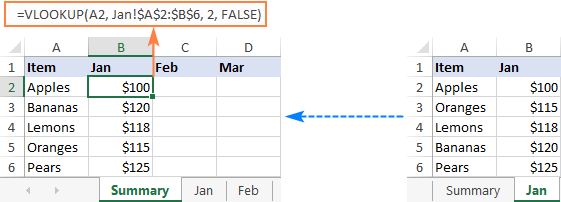In a similar manner, you can Vlookup data from the Feb and Mar sheets:

`=VLOOKUP(A2, Feb!\$A\$2:\$B\$6, 2, FALSE)`

`=VLOOKUP(A2, Mar!\$A\$2:\$B\$6, 2, FALSE)`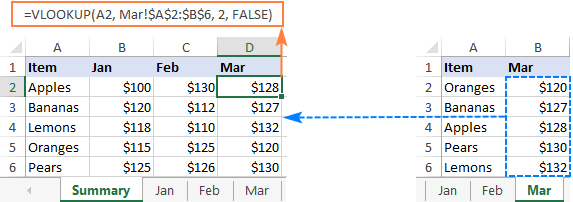Tips and notes:

• If the sheet name contains spaces or non-alphabetical characters, it must be enclosed in single quotation marks, like 'Jan Sales'!\$A\$2:\$B\$6. For more info, please see How to reference another sheet in Excel.
• Instead of typing a sheet name directly in a formula, you can switch to the lookup worksheet and select the range there. Excel will insert a reference with the correct syntax automatically, sparing you the trouble to check the name and troubleshoot.

## Vlookup from a different workbook

To VLOOKUP between two workbooks, include the file name in square brackets, followed by the sheet name and the exclamation point.

For example, to search for A2 value in the range A2:B6 on Jan sheet in the Sales_reports.xlsx workbook, use this formula:

`=VLOOKUP(A2, [Sales_reports.xlsx]Jan!\$A\$2:\$B\$6, 2, FALSE)`

For full details, please see VLOOKUP from another workbook in Excel.

## Vlookup across multiple sheets with IFERROR

When you need to look up between more than two sheets, the easiest solution is to use VLOOKUP in combination with IFERROR. The idea is to nest several IFERROR functions to check multiple worksheets one by one: if the first VLOOKUP does not find a match on the first sheet, search in the next sheet, and so on.

To see how this approach works on real-life data, let's consider the following example. Below is the Summary table that we want to populate with the item names and amounts by looking up the order number in West and East sheets: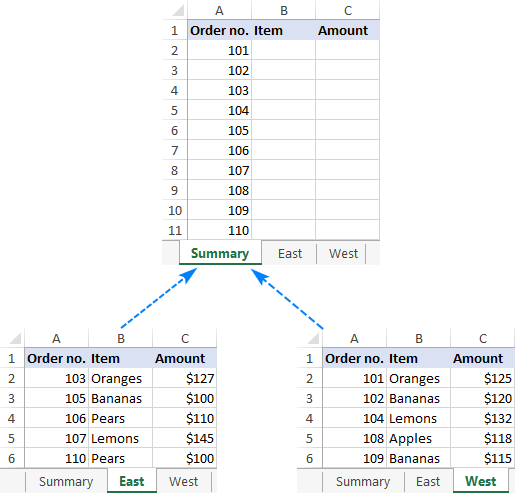First, we are going to pull the items. For this, we instruct the VLOOKUP formula to search for the order number in A2 on the East sheet and return the value from column B (2nd column in table_array A2:C6). If an exact match is not found, then search in the West sheet. If both Vlookups fail, return "Not found".

`=IFERROR(VLOOKUP(A2, East!\$A\$2:\$C\$6, 2, FALSE), IFERROR(VLOOKUP(A2, West!\$A\$2:\$C\$6, 2, FALSE), "Not found"))`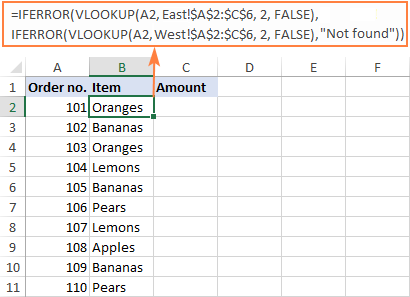To return the amount, simply change the column index number to 3:

`=IFERROR(VLOOKUP(A2, East!\$A\$2:\$C\$6, 3, FALSE), IFERROR(VLOOKUP(A2, West!\$A\$2:\$C\$6, 3, FALSE), "Not found"))`

Tip. If needed, you can specify different table arrays for different VLOOKUP functions. In this example, both lookup sheets have the same number of rows (A2:C6), but your worksheets may be different in size.

### Vlookup in multiple workbooks

To Vlookup between two or more workbooks, enclose the workbook name in square brackets and put it before the sheet name. For example, here's how you can Vlookup in two different files (Book1 and Book2) with a single formula:

`=IFERROR(VLOOKUP(A2, [Book1.xlsx]East!\$A\$2:\$C\$6, 2, FALSE), IFERROR(VLOOKUP(A2, [Book2.xlsx]West!\$A\$2:\$C\$6, 2, FALSE),"Not found"))`

### Make column index number dynamic to Vlookup multiple columns

In situation when you need to return data from several columns, making col_index_num dynamic could save you some time. There are a couple of adjustments to be made:

• For the col_index_num argument, use the COLUMNS function that returns the number of columns in a specified array: COLUMNS(\$A\$1:B\$1). (The row coordinate does not really matter, it can be just any row.)
• In the lookup_value argument, lock the column reference with the \$ sign (\$A2), so it remains fixed when copying the formula to other columns.

As the result, you get a kind of dynamic formula that extracts matching values from different columns, depending on which column the formula is copied to:

`=IFERROR(VLOOKUP(\$A2, East!\$A\$2:\$C\$6, COLUMNS(\$A\$1:B\$1), FALSE), IFERROR(VLOOKUP(\$A2, West!\$A\$2:\$C\$6, COLUMNS(\$A\$1:B\$1), FALSE), "Not found"))`

When entered in column B, COLUMNS(\$A\$1:B\$1) evaluates to 2 telling VLOOKUP to return a value from the 2nd column in the table array.

When copied to column C (i.e. you've dragged the formula from B2 to C2), B\$1 changes to C\$1 because the column reference is relative. Consequently, COLUMNS(\$A\$1:C\$1) evaluates to 3 forcing VLOOKUP to return a value from the 3rd column.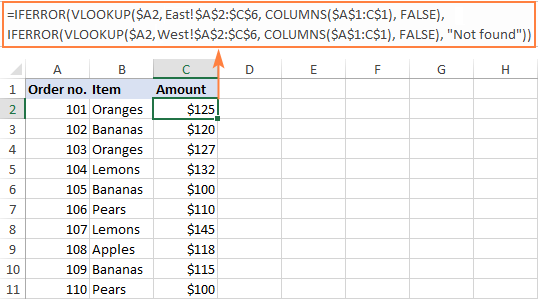This formula works great for 2 - 3 lookup sheets. If you have more, repetitive IFERRORs become too cumbersome. The next example demonstrates a bit more complicated but a lot more elegant approach.

## Vlookup multiple sheets with INDIRECT

One more way to Vlookup between multiple sheets in Excel is to use a combination of VLOOKUP and INDIRECT functions. This method requires a little preparation, but in the end, you will have a more compact formula to Vlookup in any number of spreadsheets.

A generic formula to Vlookup across sheets is as follows:

VLOOKUP(lookup_value, INDIRECT("'"&INDEX(Lookup_sheets, MATCH(1, --(COUNTIF(INDIRECT("'" & Lookup_sheets & "'!lookup_range"), lookup_value)>0), 0)) & "'!table_array"), col_index_num, FALSE)

Where:

• Lookup_sheets - a named range consisting of the lookup sheet names.
• Lookup_value - the value to search for.
• Lookup_range - the column range in the lookup sheets where to search for the lookup value.
• Table_array - the data range in the lookup sheets.
• Col_index_num - the number of the column in the table array from which to return a value.

For the formula to work correctly, please bear in mind the following caveats:

• It's an array formula, which must be completed by pressing Ctrl + Shift + Enter keys together.
• All the sheets must have the same order of columns.
• As we use one table array for all lookup sheets, specify the largest range if your sheets have different numbers of rows.

### How to use the formula to Vlookup across sheets

To Vlookup multiple sheets at a time, carry out these steps:

1. Write down all the lookup sheet names somewhere in your workbook and name that range (Lookup_sheets in our case).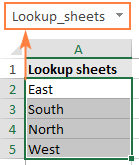2. Adjust the generic formula for your data. In this example, we'll be:
• searching for A2 value (lookup_value)
• in the range A2:A6 (lookup_range) in four worksheets (East, North, South and West), and
• pull matching values from column B, which is column 2 (col_index_num) in the data range A2:C6 (table_array).

With the above arguments, the formula takes this shape:

`=VLOOKUP(\$A2, INDIRECT("'"&INDEX(Lookup_sheets, MATCH(1, --(COUNTIF(INDIRECT("'"& Lookup_sheets&"'!\$A\$2:\$A\$6"), \$A2)>0), 0)) &"'!\$A\$2:\$C\$6"), 2, FALSE)`

Please notice that we lock both ranges (\$A\$2:\$A\$6 and \$A\$2:\$C\$6) with absolute cell references.

3. Enter the formula in the topmost cell (B2 in this example) and press Ctrl + Shift + Enter to complete it.
4. Double click or drag the fill handle to copy the formula down the column.

As the result, we've got the formula to look up the order number in 4 sheets and retrieve the corresponding item. If a specific order number is not found, a #N/A error is displayed like in row 14: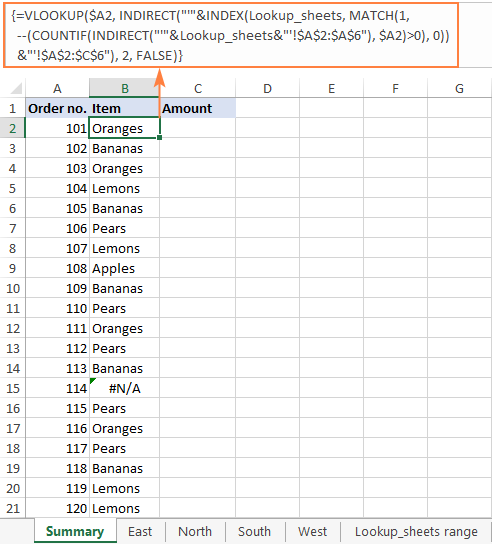To return the amount, simply replace 2 with 3 in the col_index_num argument as amounts are in the 3rd column of the table array:

`=VLOOKUP(\$A2, INDIRECT("'"&INDEX(Lookup_sheets, MATCH(1, --(COUNTIF(INDIRECT("'" & Lookup_sheets & "'!\$A\$2:\$A\$6"), \$A2)>0), 0)) & "'!\$A\$2:\$C\$6"), 3, FALSE)`

If you'd like to replace the standard #N/A error notation with your own text, wrap the formula into the IFNA function:

`=IFNA(VLOOKUP(\$A2, INDIRECT("'"&INDEX(Lookup_sheets, MATCH(1, --(COUNTIF(INDIRECT("'" & Lookup_sheets & "'!\$A\$2:\$A\$6"), \$A2)>0), 0)) & "'!\$A\$2:\$C\$6"), 3, FALSE), "Not found")`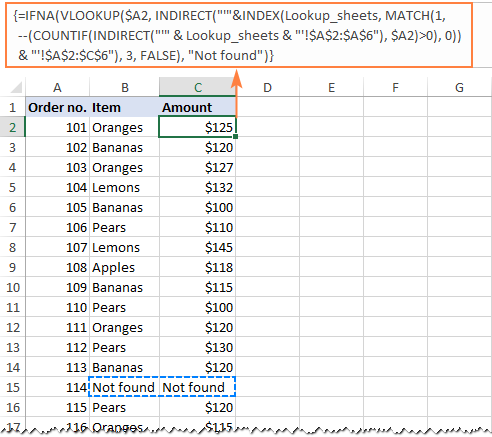### Vlookup multiple sheets between workbooks

This generic formula (or its any variation) can also be used to Vlookup multiple sheets in a different workbook. For this, concatenate the workbook name inside INDIRECT like shown in the below formula:

`=IFNA(VLOOKUP(\$A2, INDIRECT("'[Book1.xlsx]" & INDEX(Lookup_sheets, MATCH(1, --(COUNTIF(INDIRECT("'[Book1.xlsx]" & Lookup_sheets & "'!\$A\$2:\$A\$6"), \$A2)>0), 0)) & "'!\$A\$2:\$C\$6"), 2, FALSE), "Not found")`

### Vlookup between sheets and return multiple columns

If you want to pull data from several columns, a multi-cell array formula can do that in one go. To create such a formula, supply an array constant for the col_index_num argument.

In this example, we wish to return the item names (column B) and amounts (column C), which are the 2nd and 3rd columns in the table array, respectively. So, the required array is {2,3}.

`=VLOOKUP(\$A2, INDIRECT("'"&INDEX(Lookup_sheets, MATCH(1, --(COUNTIF(INDIRECT("'"& Lookup_sheets &"'!\$A\$2:\$C\$6"), \$A2)>0), 0)) &"'!\$A\$2:\$C\$6"), {2,3}, FALSE)`

To correctly enter the formula in multiple cells, this is what you need to do:

• In the first row, select all the cells to be populated (B2:C2 in our example).
• Type the formula and press Ctrl + Shift + Enter. This enters the same formula in the selected cells, which will return a different value in each column.
• Drag down the formula to the remaining rows.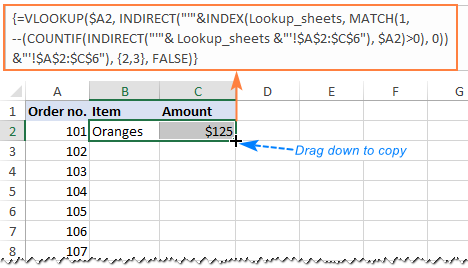### How this formula works

To better understand the logic, let's break down this basic formula to the individual functions:

`=VLOOKUP(\$A2, INDIRECT("'"&INDEX(Lookup_sheets, MATCH(1, --(COUNTIF(INDIRECT("'"& Lookup_sheets&"'!\$A\$2:\$A\$6"), \$A2)>0), 0)) &"'!\$A\$2:\$C\$6"), 2, FALSE)`

Working from the inside out, here's what the formula does:

#### COUNTIF and INDIRECT

In a nutshell, INDIRECT builds the references for all lookup sheets, and COUNTIF counts the occurrences of the lookup value (A2) in each sheet:

`--(COUNTIF( INDIRECT("'"&Lookup_sheets&"'!\$A\$2:\$A\$6"), \$A2)>0)`

In more detail:

First, you concatenate the range name (Lookup_sheets) and the range reference (\$A\$2:\$A\$6), adding apostrophes and the exclamation point in the right places to make an external reference, and feed the resulting text string to the INDIRECT function to dynamically refer to the lookup sheets:

`INDIRECT({"'East'!\$A\$2:\$A\$6"; "'South'!\$A\$2:\$A\$6"; "'North'!\$A\$2:\$A\$6"; "'West'!\$A\$2:\$A\$6"})`

COUNTIF checks each cell in the range A2:A6 on each lookup sheet against the value in A2 on the main sheet and returns the count of matches for each sheet. In our dataset, the order number in A2 (101) is found in the West sheet, which is 4th in the named range, so COUNTIF returns this array:

`{0;0;0;1}`

Next, you compare each element of the above array with 0:

`--({0; 0; 0; 1}>0)`

This yields an array of TRUE (greater than 0) and FALSE (equal to 0) values, which you coerce to 1's and 0's by using a double unary (--), and get the following array as the result:

`{0; 0; 0; 1}`

This operation is an extra precaution to handle a situation when a lookup sheet contains several occurrences of the lookup value, in which case COUNTIF would return a count greater than 1, while we want only 1's and 0's in the final array (in a moment, you will understand why).

After all these transformations, our formula looks as follows:

`VLOOKUP(\$A2, INDIRECT("'"&INDEX(Lookup_sheets, MATCH(1, {0;0;0;1}, 0)) &"'!\$A\$2:\$C\$6"), 2, FALSE)`

#### INDEX and MATCH

At this point, a classic INDEX MATCH combination steps in:

`INDEX(Lookup_sheets, MATCH(1, {0;0;0;1}, 0))`

The MATCH function configured for exact match (0 in the last argument) looks for the value 1 in the array {0;0;0;1} and returns its position, which is 4:

`INDEX(Lookup_sheets, 4)`

The INDEX function uses the number returned by MATCH as the row number argument (row_num), and returns the 4th value in the named range Lookup_sheets, which is West.

So, the formula further reduces to:

`VLOOKUP(\$A2, INDIRECT("'"&"West"&"'!\$A\$2:\$C\$6"), 2, FALSE)`

#### VLOOKUP and INDIRECT

The INDIRECT function processes the text string inside it:

`INDIRECT("'"&"West"&"'!\$A\$2:\$C\$6")`

And converts it into a reference that goes to the table_array argument of VLOOKUP:

`VLOOKUP(\$A2, 'West'!\$A\$2:\$C\$6, 2, FALSE)`

Finally, this very standard VLOOKUP formula searches for the A2 value in the first column of the range A2:C6 on the West sheet and returns a match from the 2nd column. That's it!

## Dynamic VLOOKUP to return data from multiple sheets into different cells

First off, let's define what exactly the word "dynamic" means in this context and how this formula is going to be different from the previous ones.

In case you have large chunks of data in the same format that are split over multiple spreadsheets, you may want to extract information from different sheets into different cells. The image below illustrates the concept: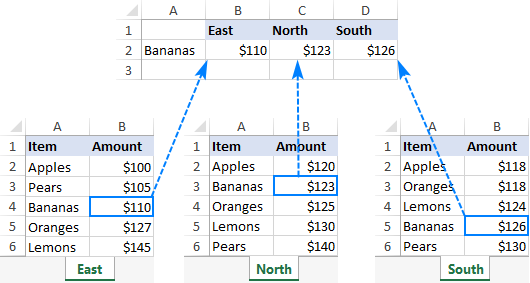Unlike the previous formulas that retrieved a value from a specific sheet based on a unique identifier, this time we are looking to extract values from several sheets at a time.

There are two different solutions for this task. In both cases, you need to do a little preparatory work and create named ranges for data cells in each lookup sheet. For this example, we defined the following ranges:

• East_Sales - A2:B6 on the East sheet
• North_Sales - A2:B6 on the North sheet
• South_Sales - A2:B6 on the South sheet
• West_Sales - A2:B6 on the West sheet

### VLOOKUP and nested IFs

If you have a reasonable number of sheets to look up, you can use nested IF functions to select the sheet based on the keywords in the predefined cells (cells B1 through D1 in our case).

With the lookup value in A2, the formula is follows:

`=VLOOKUP(\$A2, IF(B\$1="east", East_Sales, IF(B\$1="north", North_Sales, IF(B\$1="south", South_Sales, IF(B\$1="west", West_Sales)))), 2, FALSE)`

Translated into English, the IF part reads:

If B1 is East, look in the range named East_Sales; if B1 is North, look in the range named North_Sales; if B1 is South, look in the range named South_Sales; and if B1 is West, look in the range named West_Sales.

The range returned by IF goes to table_array of VLOOKUP, which pulls a matching value from the 2nd column on the corresponding sheet.

The clever use of mixed references for the lookup value (\$A2 - absolute column and relative row) and the logical test of IF (B\$1 - relative column and absolute row) allows copying the formula to other cells without any changes - Excel adjusts the references automatically based on the relative position of a row and column.

So, we enter the formula in B2, copy it right and down to as many columns and rows as needed, and get the following result: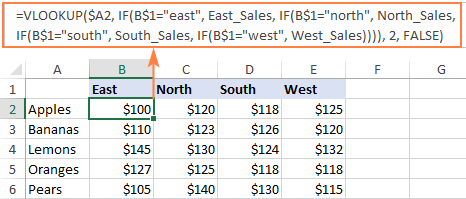### INDIRECT VLOOKUP

When working with many sheets, multiple nested levels could make the formula too lengthy and difficult to read. A far better way is to create a dynamic vlookup range with the help of INDIRECT:

`=VLOOKUP(\$A2, INDIRECT(B\$1&"_Sales"), 2, FALSE)`

Here, we concatenate the reference to the cell that contains a unique part of the named range (B1) and the common part (_Sales). This produces a text string like "East_Sales", which INDIRECT converts to the range name understandable by Excel.

As the result, you get a compact formula that works beautifully on any number of sheets: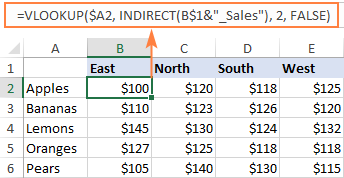That's how to Vlookup between sheets and files in Excel. I thank you for reading and hope to see you on our blog next week!

Vlookup multiple sheets examples (.xlsx file)

## You may also be interested in

1. Hello!

I am using the below formula in Google sheets, I noticed it functions in Excel but does not return the values in Google Sheets. Is there a different way this needs to be formatted to operate in google sheets? I noticed in one of the comments Import range is mentioned as a fix for google sheets. Is that what would need to be edited? if so where in the formula would this need to be added?

=ArrayFormula(VLOOKUP(\$A2, INDIRECT("'"&INDEX(LookupSheets, MATCH(1, --(COUNTIF(INDIRECT("'"& LookupSheets&"'!\$A\$2:\$A\$30"), \$A2)>0), 0)) &"'!\$A\$2:\$L\$30"), 12, FALSE))

2. I want use multiple lookup formula
Date
Shift
Lookup value
Lookup array
This is data range i want look up lookup value with match with date and shift

3. HI all
I need two table array lookups (each in a different worksheet), I've tried this to no avail. I am sure I'm missing something very obvious:

=VLOOKUP(\$A1,IF(\$B1="criteria 1),'[criteria 1 filename.xlsx]list'!\$1:\$1048576,IF(\$B1="criteria 2",’[criteria 2 filename.xlsx]list'!\$1:\$1048576)))), 2, FALSE).

Many thanks.

4. HI all
I need two table array lookups (each in a different worksheet), I've tried this to no avail. I am sure I'm missing something very obvious:

=VLOOKUP(\$A1,IF(\$B1="criteria 1),'[criteria 1 filename.xlsx]list'!\$1:\$1048576,IF(\$B1="criteria 2",’[criteria 2 filename.xlsx]list'!\$1:\$1048576)))), 2, FALSE).

Many thanks.

5. Hi,
I used the IFERROR nested function but It says I used the maximum amount of IFERROR in one formula, but I still had more sheets in a workbook. What should I do?

• Hi!
I can't see your formula, so I can only guess. Microsoft doesn't say anything about the IFERROR usage limit. For the IF function, the usage limit is 64. Your formula may have exceeded 255 characters.
Here is the article that may be helpful to you: Best practices for using IFERROR in Excel

6. i need vlookup formua for multiple sheets
sheet1 - data1(numbers)
sheet2- data price
sheet3- that data ids
sheet4- wana apply all these while taking data1 the remaining sheets also wanna visible on this
plz if you have this type formulae will you plz share that formula excel on my mail id

7. How do I use vlookup from 4 different tabs to pull data into Master tab in the same excel

• Hi!
See above - VLOOKUP multiple sheets with INDIRECT

8. Dear. I am using this formula, it work for Excel with multiple sheet, however, it got some error in google sheet

=ARRAY_CONSTRAIN(ARRAYFORMULA(ROUNDUP(VLOOKUP(\$E11, INDIRECT("'"&INDEX(Lookup_sheets, MATCH(1, --(COUNTIF(INDIRECT("'"& Lookup_sheets&"'!\$A\$2:\$A\$1000"), \$E11)>0), 0)) &"'!\$A\$2:\$P\$1000"), 12, FALSE),3)), 1, 1)

The lookup_sheet (named ranged)
1. Kitchen
2. Show Kitchen
3. Bakery

from three above, excel work it and able to find the value. However, when perform in google sheet, the indirect unable to look for the value, the lookup value only able to find the first row of named ranged which is KITCHEN. May i know any solution on this?

• Hello Reeve,

To get help with your formula, consider sharing an editable copy of your Google spreadsheet (not Excel) with us (support@apps4gs.com). To grant us access to your data, press the Share button at the upper right corner of your Google Sheets and enter support@apps4gs.com. Let us know where the formula is and we'll look into it.

Note. We keep that Google account for file sharing only and don't monitor its Inbox. Please do not email there. Once you share the file, just confirm by replying to this comment.

9. i have a query. i have a sheet with all the roll numbers and students of a class. i have another sheet which have five test marks of students only who have given exams. i have to now copy these marks in in front of respective roll numbers in first sheet. how can i do it. becausew in any second sheet many roll no. s entries are missing as they havent' given exam.

10. I am trying to combine a double Vlookup function with and IMPORTATRANGE function. Basically:

1)

2) This is where I get stuck...
I want to do a second vlookup that will in a way filter the data according to the view of the Month that I select in Cell B2 (January, March, or April). I don't want to add more columns, I just want columns B and C to display the data.

I figured using a double vlookup + an Importrange function would work, not sure if there is an easier way. Can you please help me?

• Hello Jose,

I replaced the third argument of your VLOOKUP with the MATCH function to find the number of the column required based on B2 contents:

11. I have an Excel file with ~250 worksheets; each worksheet has a unique name (the first and last name of an employee). We asked our employees a series of 10 questions. On every sheet, column C is a "yes" column; column D is a "no" column. If they answered "yes", we entered a 1 into Column C on their worksheet; if "no" we entered a 1 in column D. I am trying to determine how to summarize the total number of employees that answered yes (Column C) or no (Column D) from all 250 work worksheets. Is there a way for me to query/lookup/reference the data without manually linking each of the 250 worksheets (shift, select cell C9 (yes), or shift, select cell D9 (no) for all 250 worksheets?

• Hello!
Here is the article that may be helpful to you: 3-D reference in Excel: reference the same cell or range in multiple worksheets
If you want to sum the same cell from all sheets except the current one, just enter a formula like this:

=SUM('*'!C9)

The sign * serves as a wildcard, which is interpreted as “all sheets except this one”. When you press Enter after entering this formula, Excel converts the formula to use the actual sheet names.
We have a ready-made solution for your task. You can consolidate data from multiple worksheets with Consolidate Sheets. It is available as a part of our Ultimate Suite for Excel that you can install in a trial mode and check how it works for free.

12. thank youuuuuuuuuuuuuuuuuuuuuuuuuuuuuuuuuuuuuuuuuuuuuuuuuuuuuuuuuuuuuuuuuuuuuuuuuuuuuuuuuuuuuuuuuuuuuuuuuuuuuuuuu very much

13. Hi,

I am looking to drag a vlookup formula from column C to to column CK with =VLOOKUP(\$A\$4,NEW!\$A:\$CJ,3,0) but I cant get the col_index_num to change as I drag (I want it to go from 3 to 4 to 5 etc as I drag along from left to right)

thanks

• Hello!
If your formula was written in column C, try using the COLUMN() function instead of 3 :

=VLOOKUP(\$A\$4,NEW!\$A:\$CJ,COLUMN(),0)

14. i need to create a excel template that uses vlookups and conditional formatting.
Backstory: Team coaches need to analyze an employees performance based on a extract pulled daily. this extract is a little confusing and hard to analyze so i want to create a template that will automatically put the data into a table and have conditional formatting based on my needs. im using vlookups to pull the data however when a new sheet is introduced , that formulas become invalid. is there anything for this?

15. Hello Alexander,

Very nice and useful article. It really helped me to reduce my length of my formula.
I applied formula to lookup values from multiple excel sheet, however considering total records and array size indirect function is taking too much time to refresh.
Can you please advise any non-volatile function / any other alternate way to improve file performance.

Below is my formula for reference -
{=VLOOKUP(\$A2, INDIRECT("'"&INDEX(Lookup_Sheets, MATCH(1, --(COUNTIF(INDIRECT("'"& Lookup_Sheets &"'!\$A:\$BA"), \$A2)>0), 0)) &"'!\$A:\$BA"), MATCH(B\$1, Column_List, 0), FALSE)}

Lookup_Sheets : is defined name for list of my excel worksheets
Column_List : is defined name for column header, column header are same in all worksheets

16. Hi,

I need to returns a value based on the sheets the matching data is found:
On the delete sheet = Delete
On de diregard sheet = Disregard
On the acton sheet = Acton
ETC. up to +/- 10 sheets

If no data is found in any sheet, the value "keep" should be set.

I started with this, (I fished somewhere on the net), it works to compare with one sheet, I need it to continue to lookup the other sheets:
=IF(ISERROR(VLOOKUP(J4;DeleteList!\$A\$1:\$A\$34974;1;FALSE));"Keep";"Delete")

After reading your article, I got this to work, but it does not give the expected result, I don't need it to return the value found, or to tell me it did not find the value + to continue to the next sheets:

I plan the use that as a filter afterward with a pivot table.

I hope I was a bit understandable.

Thank you
Br,

• Hello!
If I understand your task correctly, the following formula should work for you:

I'd recommend you to have a look at our Ablebits Data - Compare multiple sheets tool that can help you. It is available as a part of our Ultimate Suite for Excel that you can install in a trial mode and check how it works for free.

• Thank you Alexandre,

This is works!

On last question, this would be a must:
The rawdata is an import and in the concerned cell (J column), for some reason an apostrophe is set in front " 'data ";
But I copy towards the concerned sheets from out the pivot table;
Because of that your formula was not working at first as it is looking for an exact match:
" data " not equal to " 'data " = not found.

Is there a way around this?
The data are unique numbers (8 numbers 12345678), if this helps

I have no admin right to install your trial "Ablebits Data", but I look it up on my personal computer, and submit it to our IT departement if I find a use for it.

Thanks again.

• Hello!
This problem often appears when importing data from another program. It is necessary to convert numbers written as text into numbers. To do this, use the guidelines from this article or the Convert Text tool from the Ultimate Suite for Excel.

17. I used the formula above to pull data from one spreadsheet to another but it's not working. It just shows my formula in the cell. However, in the "Function Arguments" box it shows the information for the first person on the sheet I'm trying to pull the data from. Can you tell me what I'm doing wrong?

• Hi Kevin,

When a cell displays a formula instead of the calculated value, most likely it's because of one of the following reasons:
- Show Formulas mode is turned on, or
- A formula is entered as text

18. I am using Google Sheets I want to change the following formula so that it can search data from another worksheet:
=IFERROR(INDEX(\$AL\$2:AL,MATCH(1,(\$AJ\$2:AJ=B6)*(\$AK\$2:AK=D6),0)))

This works if I maintain all of my data in the same sheet but my Zapier automation requires me to transfer columns AJ,AK,and AL from the previous formula to another worksheet. There AJ=A, AK=B, AL=C. I tried using the following formula because I don't want to import data into my current worksheet other than that which is required:
=IFERROR(INDEX(\$AL\$2:AL,MATCH(1,((VLOOKUP(B5,'Info de otras hojas'! \$A\$2:A,1,FALSE*(VLOOKUP(D5,'Info de otras hojas'! \$B\$2:B,3,FALSE))),0))))).
Also, I'm not sure about how to include the first part after INDEX in VLOOKUP.

• Hello Irving,

to do vlookup from a different spreadsheet in Google Sheets, you need to embed the IMPORTRANGE function in the range arguments.

19. I have a working version of a Vlookup across multiple sheets with INDIRECT but I'm trying to include a 'Choose' function so I can specify a custom range to lookup values where the key isn't in the first column E.g. From this:

=VLOOKUP(\$B9;INDIRECT("'"&\$A9&"'!\$A\$14:\$AR\$45");2;FALSE)

to this:

=VLOOKUP(\$B9;INDIRECT("'"&\$A9&"'!CHOOSE({1:2},D14:D45,B14:B45)");2;FALSE)

I can't figure out if a - it's even possible to combine these two and b - if I have a syntax issue given I've changed the value of B9 in line with the value I want to search for in B14:B45 across the other sheets.

• Hello!
Without seeing your data it is difficult to give you any advice. If the search key is not in the first column, I recommend using the INDEX + MATCH functions. Here is the article that may be helpful to you: INDEX & MATCH in Excel - better alternative to VLOOKUP
If this is not what you wanted, please describe the problem in more detail.

20. Hello,
please trying to use the Vlookup function in a excel data sheet but having some challenges.
the challenge is that; after writing the logic for the function in a cell and running it down to cover the data, some cell return with #N/A or different corresponding infomation but when i copy the figure am trying to vlookup for in the data i find the figure in the data.

any help?

21. I have two or three instances of excel open. I needed to do this because my workbooks are very large and they keep crashing (recent issue... anyone else seeing this?). Doing this however, does not allow me to VLOOKUP across instances... Is there a way to do this?

22. Hi! Thank you for your explanaiton. I've modified the formula to reference tables instead of sheets, and use xlookup instead of vlookup, and it looks like this:
=XLOOKUP([@Key],INDIRECT(INDEX(Tablas,MATCH(1,--(COUNTIF(INDIRECT(Tablas&"[Key]"),[@Key])>0),0))&"[Key]"),INDIRECT(INDEX(Tablas,MATCH(1,--(COUNTIF(INDIRECT(Tablas&"[Key]"),[@Key])>0),0))&"[Estatus en Nomina]"),"")

However, when I run the formula I get this error:
COUNTIF({#value!;#value!;#value!;#value!;#value!},[@Key])
It should give me 0 for all of them, but it turns into
--({0;0;0;1;0}>0

thus giving me the wrong value in the end. How can I fix this?
Hope this was clear enough. Thanks again!

• NVM found my mistake :) thank you!

• Hi Alejandra, are you able to share your solution to this? I really need to use XLOOKUP and cannot figure out how to change the original formula.

23. Thank you so much Alexander for your reply but I may not have explained what I want to achieve clearly enough.

I want to do exactly the same thing as described in the following link except the data is in different whorksheets. I want to lookup multiple values which appear multiple time and are in mutliple worksheets and return the data from corresponding columns into another worksheets.

Many thanks

• Hello!
This is a complex solution that cannot be found with a single formula.
Unfortunately, without seeing your data it is impossible to give you advice.
I'm sorry, it is not very clear what result you want to get. Could you please describe your task in more detail and send us a small sample workbook with the source data and expected result to support@ablebits.com? Please shorten your tables to 10-20 rows/columns and include the link to your blog comment.

24. Hi. I'd like to start by commending you on your tutorials on this site. They've been extremely helpful and have become invaluable.

However, although I have found a tutorial on looking up multiple matches based on mutliple conditions this only shows how to do this within the same worksheet. Also whilst the tutorial above shows how to look up values accross worksheets and workbooks I can't see it shows how to get multiple matches.

So is it possible to lookup and return multiple matches accross multiple worksheets and if so how?

• Hello!
In the COUNTIF function, use links to other sheets in the workbook.
I'd recommend you to have a look at our Ablebits Data - Compare tables tool that can help you to find duplicates in two tables.
It is available as a part of our Ultimate Suite for Excel that you can install in a trial mode and check how it works for free

• Thank you so much Alexander for your reply, however, maybe I didn't explain what I'm trying to do clearly enough.

I want to lookup values which appear more than once in a table and retun data from a corresponding column just like this

Except I want to lookup more than 1 value each of which appear more then once in each table and these tables are in different sheets and then return data from a corresponding column in the same tables. Exactly as described in the link above but the data to be looked up is in different worksheets to that where the formula is to be entered.

Many thanks.

• Have you ever gotten an answer for this?

26. Hello.. Alexander,

I have a problem regarding vlookup and then sum for values which are came by vlookup. as example there is data in cell A1,C1 and E1.
By using vlookup we call values from other sheet to B1,D1 and F1 respective to A1, C1 and E1. Now we take sum of B1,D1 and F1 to cell G1.

Now problem is that if there is any empty cell in column A,C or in E the value will not came in respective cell by vlookup and the sum function shows #value error in column G.

A. B. C. D. E. F. G.
1. 001. 2. 002. 2. 003. 4. 8.
2. 004. 2. 005. 3. 006. 1. 6.
3. 007. 1. 008. 3. EMPTY N/A. #VALUE

well, im working on old data sheet and there is 8 columns like this and some other data))..
I tried some formulas but not getting result. Is there any function that ignore empty cell and calculate only that cell which contains value from vlookup.

• Hello!
Enclose the VLOOKUP function in the IFERROR function to return 0 or "" instead of an error.

• In short, is there any other options for this?

=IF(V1="",IF(S1="",IF(P1="",IF(M1="",IF(J1="",IF(G1="",IF(D1="",IF(A1="","",A1),+A1+D1),+A1+D1+G1),A1+D1+G1+J1),+A1+D1+G1+J1+M1),+A1+D1+G1+J1+M1+P1),+A1+D1+G1+J1+M1+P1+S1),+A1+D1+G1+J1+M1+P1+S1+V1)

27. I have a workbook showing an employee list and a breakdown of their hours worked for each week. A tab will be added each week; this workbook also has a summary sheet, to compile the info from all tabs. I am currently using a VLOOKUP formula, but it has to modified each time a tab is added. This is being done across 15 columns, so updating the formula is a bit cumbersome. Is there a better way to accomplish this? Could the VLOOKUP look at a range of sheets (the layout is the same for each sheet), with new tabs being inserted within that range to avoid having to update the formula each week?

Example: Employee names are listed in column A, but may not be in the same row, in every sheet; the breakdown of time is across columns C - N (ie, Training, Holiday, Jury Duty, Regular). This layout is the same in each sheet, including the Summary.

My formula to summarize Holiday hours for the first employee on the list looks like this:
=VLOOKUP(\$A10,'TOTAL HRS SHEET 01-02'!\$A:\$J,7,FALSE)+VLOOKUP(\$A10,'TOTAL HRS SHEET 01-09'!\$A:\$J,7,FALSE)+VLOOKUP(\$A10,'TOTAL HRS SHEET 01-16'!\$A:\$J,7,FALSE)+VLOOKUP(\$A10,'TOTAL HRS SHEET 01-23'!\$A:\$J,7,FALSE)+VLOOKUP(\$A10,'TOTAL HRS SHEET 01-30'!\$A:\$J,7,FALSE)+VLOOKUP(\$A10,'TOTAL HRS SHEET 01-31'!\$A:\$J,7,FALSE).

This is just January. It works, but is there a better way?

• Hello!

28. I'm trying to use a portion of a cells contents as a wildcard search to then return the value from a different worksheet that has the matching value of the wildcard search from the lookup_value. Is this possible?

I tried using VLOOKUP("*"&lookup_value&"*",worksheet array,value needed,FALSE) but it is not finding anything.

• Hi,
The information you provided is not enough to understand your case and give you any advice, sorry. Unfortunately, without seeing your data it is difficult to give you any advice. Please provide me with an example of the source data and the expected result. Please specify what formula you used and what problem or error occurred. It’ll help me understand it better and find a solution for you.

29. I am new to excel. Dealing purely with numbers and some letters. I have multiple worksheets with data in the same workbook maybe 15 to 30 worksheets. What I want appears simple to me. I need to know how many cells have the same content wanting excel to return the exact number of cells and where they are. For example a cell might have E3 F12 G10 in it and appear 20 times in different locations say worksheet called "Table 5" and another called Table 17" etc or if in the same worksheet but different column like cell "B55230" and the other cell "F20456" in column F. Please let me know if the countif with vlookup functions can help.

• Hello!
This task is too difficult for the VLOOKUP function. We have a ready-made solution for your task. I'd recommend you to have a look at our Ablebits Tools — Duplicate remover.
You can install in a trial mode and check how it works for free.

30. I am trying to add lexile values from teenbiz to compare lexile values in NWEA

• Hi,
Sorry, it's not quite clear what you are trying to achieve. For me to be able to help you better, please describe your task in more detail. Please specify what you were trying to find, what formula you used and what problem or error occurred. Give an example of the source data and the expected result.
It’ll help me understand it better and find a solution for you.

31. Hi! Thank you for this! I am not sure if this is the right place for this. I am trying to find a specific formula. My workbook has several worksheets of data. Each worksheet represents a year worth of data. On the first page we are analyzing the overall data and showing the overall average, as well as which years have the max and min of certain stats. I have figured that out. But I want to figure out how to display on the first sheet, which sheet that value came from. One of the tricks is that we are not comparing the same cell in each sheet because we have a different number of transactions from year to year.

For example, this is on of the Max formulas:
=MAX('2020'!B61,'2019'!B46,'2018'!B45,'2017'!B41,'2016'!B37,'2015'!B39,'2014'!B35,'2013'!B41)

• Hello!
I recommend creating a separate table with totals from each sheet. In this table it will be easy to find the maximum value and determine the year.

32. I have used VLOOKUP over 2 sheet previously without any problems.
Today though, it's telling that I have entered to few arguments for this function. I even used formula tab to make sure that all is filled in.
=vlookup(D5 '2021Tarrif'!A5:I59 4 0)
is there something that I'm not doing right?

• Hello!
You wrote down the formula without commas.

=VLOOKUP(D5, '2021Tarrif'!A5:I59, 4, 0)

You are using your unique links, I do not have your data. Therefore, I cannot check the work of the formula. If you tell me what the problem is and what the error is, I will try to help.

33. I'm ridiculously new to excel and with every task I need to look up a formula. My question is: I have two sheets (employees information sheet 1, employee participation sheet 2--it's A LOT of data. I need to find an exact match of an ID on sheet 2 to to sheet 1 and if sheet 1 contains the match, to the right of that match on I need the value to be yes... So what is best?? VLOOKUP or INDEX/MATCH.. I've read everything and I think I've spiraled into an abyss...and when I try what I think is the formula it doesn't work... I'm hopeless.

34. {=vlookup(A5,sheet1!\$B\$2:\$D\$269,3,0)}
Pls tell me what is the mistake in this formula still the value is not found

35. Thank You

36. I am Chandresh,
Thanks for this information about vlookup.
I am new for this and try to learn.
I have problem and I want to help from you.
I have a data in 'Data' sheet with column's name- date,item no,item,unit and planned, second sheet 'Planned' which has vertical data- item,cum,item no & horizontal data -
Date(day to day).
Now I want save my values from 'Data' sheet to 'planned' sheet automatically match to item and day by day.
Pls help

37. I have a given formula that pulls the scores from another tabsheet. Summary tabsheet and Assessment tabsheet.

On Summary tabsheet, I have this given formula.
=IFERROR((VLOOKUP(\$Y14,'Pre-SAx Assessment'!\$E\$2:\$J\$8225,6,FALSE)),"0")

where Y14 is the value used for search

There are multiple repeated scores from the "Assessment" tabsheet in column J. Without sorting the Assessment tabsheet table, it just return the first value it found. How can I improve on this given formula using the 'max' option within?

• Hello!
If I understand your problem correctly, you want to find the last match. This is not possible with VLOOKUP. I recommend using the LOOKUP function and these examples.
I hope this will help, otherwise please do not hesitate to contact me anytime.

38. Oh it helped me out soooooooooo much! Thanks a bunch! :) :D

39. I have implemented this into a Google Sheets document that I am working on. However, it doesn't return any values from any sheets but the first listed in the named range. This could be a Google sheets issue, or I'm missing something...

• Hi Sean,

Google Sheets syntax is a bit different. Make sure you reference other sheets correctly. Svetlana provided examples for spreadsheets in this special blog post.

• Thanks for your reply, Natalia. I compared the reference you provided to my formula, and I don't see a difference in the syntax. If you have a moment, I'd love to allow you to take a look at my sheet and see if you can discern the issue. I've exhausted different tweaks in the formula, as well as the datasets. No matter what, I can't get the calculations to advance to the next sheet in the named range.

40. Thanks it solve my problem.

41. Nice article !

42. This is working good for me vlookup value for multiple sheets
=VLOOKUP(\$A2, INDIRECT("'"&INDEX(Lookup_sheets, MATCH(1, --(COUNTIF(INDIRECT("'"& Lookup_sheets&"'!\$A\$2:\$A\$6"), \$A2)>0), 0)) &"'!\$A\$2:\$C\$6"), 2, FALSE)
at the same time i want cell reference " i want cell address instead of value)
=CELL("address",VLOOKUP(\$A2, INDIRECT("'"&INDEX(Lookup_sheets, MATCH(1, --(COUNTIF(INDIRECT("'"& Lookup_sheets&"'!\$A\$2:\$A\$6"), \$A2)>0), 0)) &"'!\$A\$2:\$C\$6"), 2, FALSE)) this is not working for me please suggest me actually i want cell address accross multiple sheets.

• Hello!
To return the address of the cell in which the desired value is written, you can use the formula:

B2 - the value we are looking for
F1: F21 - search range
6 is the column number of the search range (that is, column F)

• i know this formula i want multiple worksheets like this =VLOOKUP(\$A2, INDIRECT("'"&INDEX(Lookup_sheets, MATCH(1, --(COUNTIF(INDIRECT("'"& Lookup_sheets&"'!\$A\$2:\$A\$6"), \$A2)>0), 0)) &"'!\$A\$2:\$C\$6"), 2, FALSE) but i want cell address instead of value

• Hello!
Without seeing your data, it is impossible to understand your formula. However, I can tell you for sure that the VLOOKUP function can never return you a cell address. It only returns the cell value.

43. I want to search between different sheets with the vlookup command, so that the name of each sheet is written inside a cell and is dynamic, that is, whenever the cell name is changed to a sheet name, a search is performed inside that sheet.
Thankful

44. I am trying to link an insurance rate table (very simple just- age and rate--two columns) sheet with the primary spread sheet with the actual age of each person to determine the rate they will pay. I am using the VLOOKUP as follows =VLOOKUP(\$I\$2,Rate!\$A\$1:\$B\$100,2,FALSE) How can I link the actual age of the person on the primary sheet to secondary sheet with the table so the rate will show on the primary sheet?

• Hello Cynthia!
Your formula is spelled correctly. But how it works with your data, I do not know. Explain in more detail what kind of problem arises. Please let me know in more detail what you were trying to find, what formula you used and what problem or error occurred. In that case I will try to help you.

45. Hi,
I am trying to use VLook up or Xlookup to connect two work sheets and having issues. I want the worksheet to find an ingredient and link the ingredient from one column to the measurement in another column. SO worksheet 3:

Xlookup("CHIA SEEDS", worksheet1!A1:A22,worksheet1!B1:B22)

But its not working for me, I want to be able to look up a row in my ingredients column on spread sheet one and link it to a customer on my customer column and have that link to work sheet 2 (if that makes sense) I also want to be able to add new rows and columns to worksheet 1 without messing up my formulas in other worksheets (so using words as the search cretira to find the measurement numbers?)

Not sure if any of this makes sense but any help is greatly appreciated!

Kindly,
Anna

• Hello Anna!
I used your formula in my worksheet. She works. It extracts the value from column B. You probably wanted to get some other result. But I could not understand which one.
I also recommend converting your worksheet1! A1: A22 and worksheet1! B1: B22 columns to named ranges or to a table, as described in the article above. This will allow you not to worry that your links will break when adding data.
For me to be able to help you better, please describe your task in more detail. Please let me know in more detail what you were trying to find, and what problem or error occurred. It’ll help me understand it better and find a solution for you. Thank you.

46. HI,
You had a lot of great article 1st of all.
I'm trying to used the Vlookup function with multiple criteria and SUM them.
Example
Row1 Product A / Jan 2020 / 8 units
Row2 Product A / Jan 2020 / 10 Units
ROw3 Product A / Jan 2020 / 5 units
The formula should count if the criteria are Product A and Jan 2020 -> 22 Units
I tried =SUM(VLOOKUP(A2&"-"&B2,A1:C3,2,FALSE)) and it's not working any idea??
THanks a lot
Regards

• Hello!
If I understand your task correctly, the following formula should work for you:
=SUMPRODUCT(--(\$A\$1:\$A\$7="Product A"),--(\$B\$1:\$B\$7="Jan 2020"),C1:C7)

47. How to do hyper link for multiple sheet

48. hi. my name is janak. thanks for the tutorial, it helps a lot.
can you explain me the concept of Vlookup multiple sheets with INDIRECT ?
it contains lots of formulas which make me confuse while evaluating it.
can you send me the excel sheet with formula & data regarding that concept & evaluation process.

• Janak,

You can find the detailed explanation of the formula in the "How this formula works" section. And here is the sample worksheet with all the formulas discussed in this tutorial.

49. That is very helpful, bit I am struggling with is how to apply VLOOKUP in only one sheet and return multiple columns if Value I am looking for is in multiple places within row A in that sheet?
Example: I have list of transactions and values one under the other. In row A I got the name of the project that transaction should be allocated and now I have created new sheet and entering formula:
=VLOOKUP(\$A\$1, Sheet1!A:A, {2,3,4,5}, FALSE)
Is there any way for excel to pick up the values in same column and then list them in new sheet made for this Value?

• Hi Milosz,

I do not clearly understand exactly what you are trying to achieve, but your formula won't work anyway - your table_array consists of a single column A while you are requesting to return values from columns 2, 3, 4, and 5.